# Tableau Scaffolding Dates – Calculating Deferred Revenue

//

There are a small number of use cases where Tableau scaffolding is the solution. In Tableau, scaffolding dates is used to “fill in” missing dates, where these dates actually need to exist in the data source. For example sometimes each date, even those not in the data, need a value for additional calculation.

This article will show two techniques to scaffold data in Tableau:

A real-world example of this is calculating deferred revenue in Tableau. To calculate deferred revenue we need a value for each date, hence this is a perfect use case for scaffolding with dates in Tableau.

Firstly, what is deferred revenue? In simple terms, it’s an accounting principle where, when delivering a service (or can be a product), instead of booking the revenue on the day received, this revenue is split over the length of the service. However, I’m not an accountant so look here for a more official definition.

Generally, to calculate deferred revenue, the revenue is calculated daily. For example, imagine a subscription costing £365 starting on 1 Jan 2019 and finishing 31 Dec 2019 inclusive. There are 365 days in 2019, so £365 / 365 days = £1 revenue per day. Therefore the deferred revenue for this product is £1 per day which aggregates to £28 for Feb 2019, £30 for Sep 2019, £365 for 2019, etc.

To make this calculation in Tableau we need a record for every date. The problem is that, in general, data isn’t stored in this way. In many systems it would contain a Contract ID, Start Date and End Date. So, using the above example, there isn’t a record for every, there are only 2 dates in the data, the 1 Jan and 31 Dec.

Therefore, to calculate the deferred revenue, we use a scaffold data source. A scaffold data set, in this case, is another set of data containing all possible dates.

## Calculate deferred revenue in Tableau

This article will show you how to calculate deferred revenue using two techniques:

1. a join to the scaffolding data set
2. using a data blend to the scaffold data

For the revenue data I’m using a dummy date set provided in a question on the Tableau forum.

To start, with either technique, first make sure Tableau recognises your data as a date data type.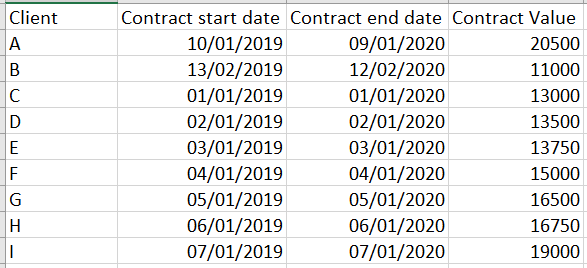These are the items to defer over their length

## Tableau date scaffolding with a join

Joining to the scaffold data source is simpler than blending. To have the option to join, the scaffold data has to be within the same data source as the revenue data. For example, either a new worksheet in the existing Excel spreadsheet data source, or a new table in a database.

As we’re going to join the date scaffold to the contract data, the scaffold data source can be kept simple. A list of dates will suffice.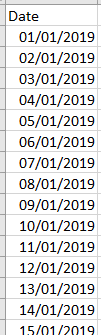Scaffold date data

This example will use Excel as the data source. Therefore add a new sheet containing the list of dates.

Now switch to Tableau. Connect to the spreadsheet. In the Tableau data source window, first add the Scaffold worksheet, then the Contracts. Next set up the join to put a value against all dates between a Start Date and End Date. Join the Date field (from Scaffold) where the date is greater than or equal to the Start Date AND where the Date field is less than the End Date.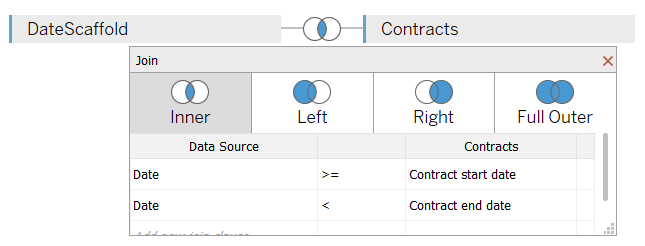How to join the Scaffold data to the Contract data

Now check the data and notice there’s a record for every date between the start and end dates. That is exactly what we need to calculate the deferred revenue.

### How to calculate the deferred revenue using a Join

If you recall, to calculate deferred revenue we need the following values:

1. Total value of contract
2. The number of days the contract will be delivered (i.e. the days between start and end dates)

The total value of the contract came in with the data, the [Contract Value] field.

#### Calculate the number of days

Use a simple DATEDIFF function to calculate the number of days:

`[Contract Days]: DATEDIFF('day',[Contract start date],[Contract end date])`

#### Calculate the revenue per day

That gives all the necessary information to calculate the daily revenue:

`[Contract Daily Value]: SUM([Contract Value])/SUM([Contract Days])`

#### Check the calculations

Now lay the data out in a simple crosstab table. This a) confirms the calculations are giving the expected totals and b) provides the target numbers to double-check the numbers stay good as fields are moved.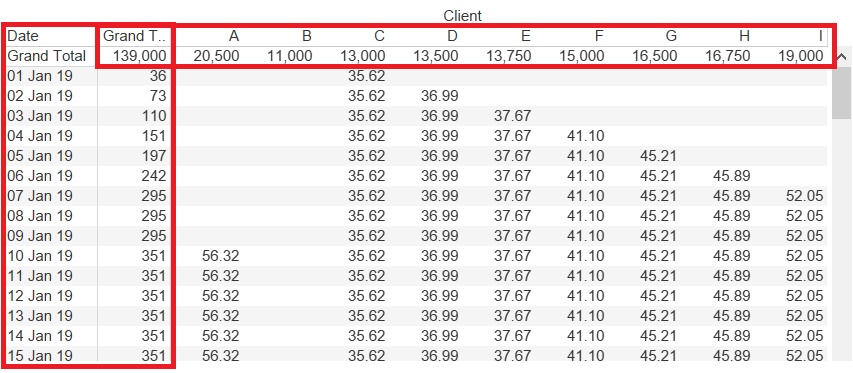The totals per day and per client are highlighted

By default the Grand Total numbers aren’t a sum of the column, but this is easy to fix. On the menu Analysis – Totals – Total All Using – Sum.

Remove the Client from the table and check the numbers are still as expected. You’ll notice they’re not. This is a matter of mathematics. With one client a contract value of £365 over 365 days = £1/day. Add a second client under the same terms, where we expect £2 per day (£1 from Client Y and £1 from Client Z). However, using our [Contract Daily Value] formula gives (£365+£365) / (365+365) = £1

Therefore, to be able to slice and dice this data, we have to calculate the value for each client for each day separately. Fortunately the introduction of the FIXED calculation means we only need a small tweak to the [Contract Daily Value] formula.

`[Contract Daily Value Fixed]: { FIXED [Client],[Date]: SUM([Contract Value])/SUM([Contract Days])}`

Using this new field for the daily value keeps the daily value regardless of how we slice and dice. For example, this is what happens when removing the client (see the total):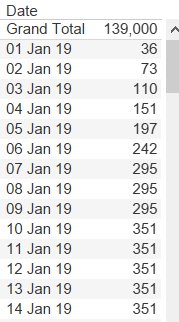Revenue per day

And this is what happens showing revenue for the year: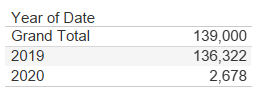Deferred Revenue per Year

#### Build the deferred revenue Tableau dashboard

Below find a dashboard showing the revenue aggregated and deferred on a monthly basis. The interactive version is at the bottom of the article.

Download the workbook from Tableau Public to see how to construct both the join and blend dashboards

## Tableau date scaffolding with a blend

It’s also possible to use Tableau data blending instead of a join to scaffold the data. However it can be significantly more complex. It forces using advanced table calculations and blending introduces other limitations. It also reduces flexibility; the table calculation settings depend on the data within the view.

In addition, building the scaffold data source also becomes more complex. Within the scaffold we need a record for every date for every client. With even a small amount of clients the data source could quickly become very large.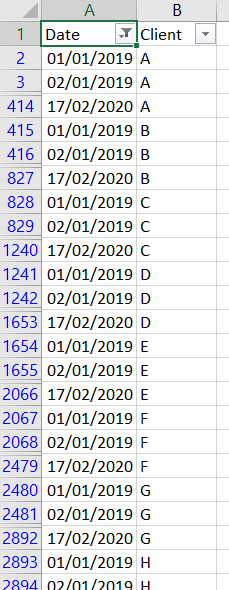All dates for all clients in the scaffold

However it is still possible. Both the Join dashboard and the Blend dashboard appear almost identical, but behind the scenes they are quite different.

### How to calculate the deferred revenue using a Blend

This time the Scaffold data is one data source and the Contract data another separate Tableau data source.

Connect the 2 data sets to Tableau and set up a relationship between them. Data – Edit Blend Relationships. Set the Primary data source as the Scaffold and the blending field as the Client.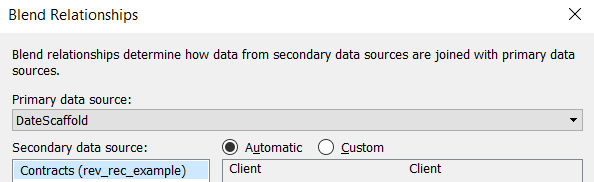Blend the data sources only on Client in the Relationships window

Be sure NOT to blend on the dates. Blending on dates can be problematic; in this case the blend will happen in a calculated field, with details below.

Almost identical to the Join example, to calculate the deferred revenue we need:

1. The contract value
2. The contract days

#### Build the calculations

All of the Dates and Clients to use in the view will come from the Scaffold. Therefore we bring the contract value into that data source. In the Scaffold data source create a calculated field:

Contract value

``````
[Value]: IF ATTR([Date])>=ATTR([Contracts (rev_rec_example)].[Contract start date])
AND ATTR([Date]) < ATTR([Contracts (rev_rec_example)].[Contract end date])
THEN SUM([Contracts (rev_rec_example)].[Contract Value])
END
``````

Effectively this joins all of the dates to the Contract Start and End dates. Very similar to the Join example, where this step was within the Join itself.

As it’s a blend and we’re pulling in fields from 2 data sources, all of the fields are aggregated. This explains the use of ATTR() for the dates.

The contract days calculation is more straightforward, it’s a DATEDIFF, calculating the number of days between this contract start and end dates. Within the Contracts data source create a calculated field:

Contract days

`[NumDays]: DATEDIFF('day',[Contract start date],[Contract end date])`

This provides enough information to calculate the revenue per day. Within the Scaffold data source create the following:

Revenue per day

`[RevPerDay]: [Value]/SUM([Contracts (rev_rec_example)].[NumDays])`

Because it’s a data blend both the Date and Client have to be somewhere within the view, even though we don’t want them visible.

#### Check the calculations

Build the view using both the Date and Client from the Scaffold data source. Add the [RevPerDay] to the view and, all being well, it will match the results of the Join scaffold.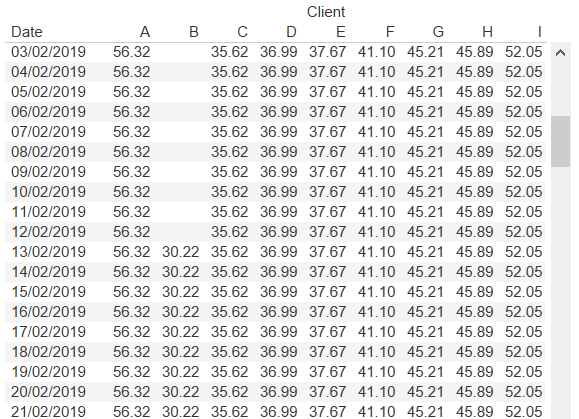The daily deferred revenue for each client

However, a blend introduces other challenges. Remove the Client from the view and see what happens. Therefore Client has to be somewhere in the view. Bringing to Detail puts it on to the view but doesn’t make it visible – but notice it still doesn’t look right. The same is also true of the Date, this also has to be in the view.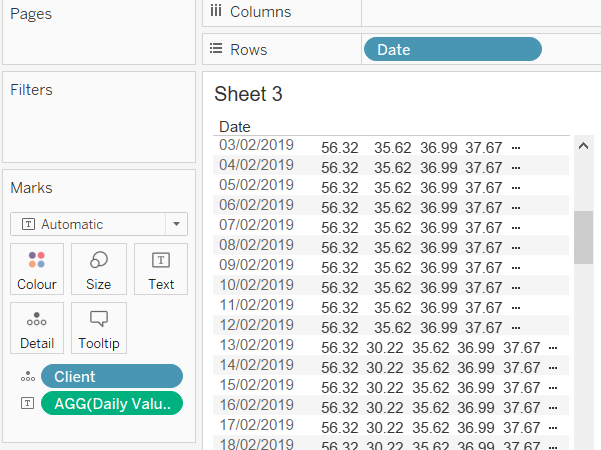Putting Client on detail impacts the numbers

This is where table calculations come into play. The current calculation shows the sum of revenue per day for every client per day (remember both Client and Date have to be in the view as these are the blending fields). We are looking for a sum of these sums and can do this with a table calculation.

#### Create the daily revenue table calculation

Use a WINDOW_SUM to convert the Revenue per day calculation to a table calculation, effectively summing the sums.

`[RevPerDay Window Sum]: IF FIRST()=0 THEN WINDOW_SUM([RevPerDay]) END`

The WINDOW_SUM simply sums all of the individual client / day values. But it does this for every mark – i.e. every occurrence of a client / day combination will share this value.

For example the entire total is 139,000 so every day/client combination would have the value 139,000…and summing that will give the incorrect totals.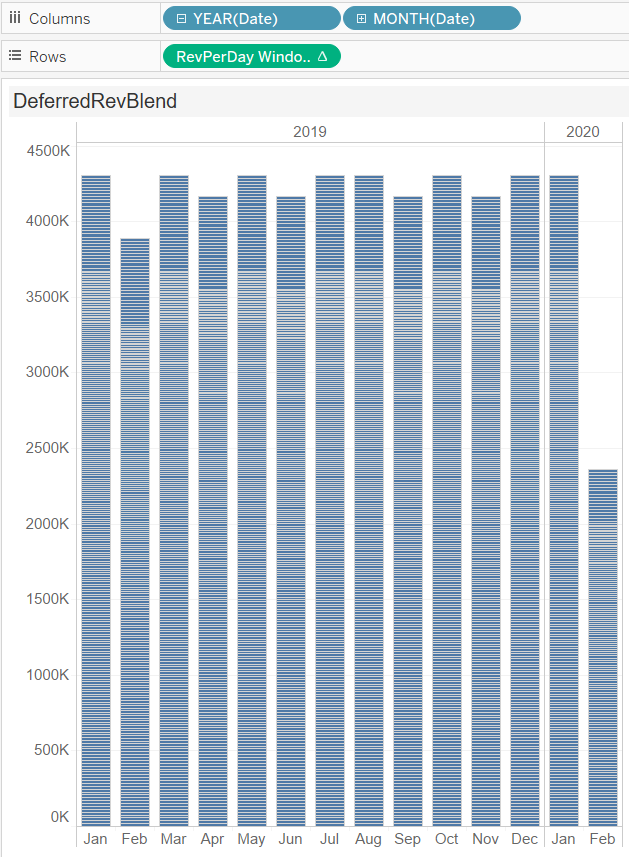Each small stripe is the result repeating leaving the revenue far higher than expected

Resolve this by using FIRST() and altering the table calculation settings. We only want one value per day so use the FIRST()=0 to keep only the first occurrence.

#### Build the dashboard

This new daily revenue calculation enables building a dashboard, as long as Client and Date are somewhere in the view.

To replicate the Join dashboard place both Client and Date on the Detail shelf. Year and Month of Date should be on Columns and the daily revenue table calculation on Rows.

That isn’t the final step; as we’re using a table calculation the advanced settings require some modification. Set as follows to restart summing every month.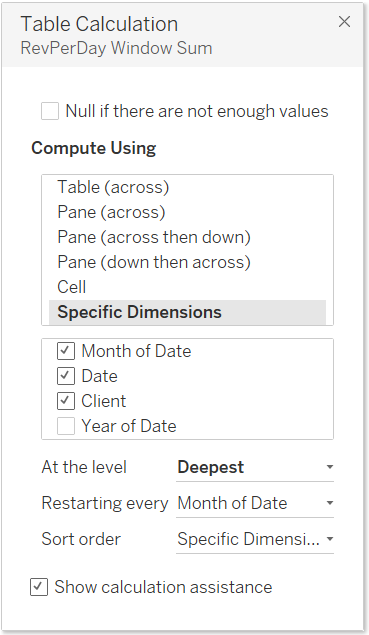Set up the Compute Using settings to return the expected result

Use a reference line to put the monthly total on top of the bar. This is a slightly different approach to that in the Join (where it is on the Text shelf), however, it does the same thing.

## Scaffolding with a Join vs a Blend

The scaffold Join and Blend versions of the deferred revenue dashboard appear almost identical. However the approach is quite different. In my opinion it is far simpler and more flexible using the join. However this isn’t always possible and it is possible with a data blend, just more fiddly!

### 1 thought on “Tableau Scaffolding Dates – Calculating Deferred Revenue”

1.Interesting blog! The way of building up a scaffold data source is explained very wel, thanks for that. Cheers Felix

Reply